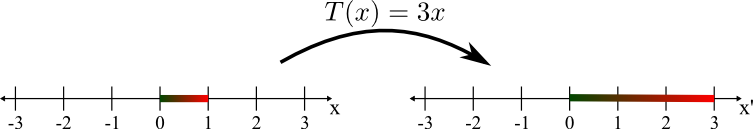# Math Insight

### Image: Stretching by the linear transformation T(x)=3xThe one-dimensional linear transformation $T(x)=3x$ maps the interval $[0,1]$ to the interval $[0,3]$. The mapping stretched the interval into a new interval of three times the original length. The red to green gradient on the intervals illustrates the mapping, as each point on the interval is mapped a point of the same color.

Image file: linear_transformation_3x.png

Source image file: linear_transformation_3x.svg
Source image type: Inkscape SVG